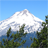# Clarification of the hash algorithm for L2 Lookup Table Index calculation on the SJA1105TEL

cancel
Showing results for
Did you mean:

## Clarification of the hash algorithm for L2 Lookup Table Index calculation on the SJA1105TEL

253 ViewsContributor II

We are trying to figure out how to to programmatically fill the L2 Address Lookup table of the SJA1105TEL switch fabric.

In order to achieve this we need to replicate the hash algorithm that is used in the switch for lookup in our software. Unfortunately the specification is not 100% clear and we can’t find example data or a sample implementation for this.

What would help us most is example code or at least data to generate the hash or index.

4.2.6 L2 Address Lookup table

Table 10

Contains the address in physical memory where this entry is stored. The physical address of an entry is calculated as INDEX = 4 x hash (MACADDR, VLANID) + i, where i Є {0;1;2;3}. If more than four MAC address/VLAN IDs pairs produce identical hashes, a different value for the POLY must be chosen or MAC addresses and VLAN IDs of endpoints or default VLANs of switch ports must be changed. If SHARED_LEARN is set, the VLANID portion of the hash computation is set to zero, regardless of the actual VLANID of the frame. The 8-bit CRC hash computation operates on 62 bits consisting of the 48-bit MAC address, 10-bit VLANID and 4 bits padded with 0.

We have tried to implement this as specified and are not getting working hash values. The following points are unclear or possibly wrong:

10-bit VLANID: VLAN IDs are 12 bit and its not clear where these 10bit come from. Are they a subset of the actual VLAN ID or is this wrongly specified and it’s actually 48bit MAC + 12bit Vid + 4 bit padding = 64bit?

Some more information can be found in:

4.2.14 L2 Lookup Parameters

This parameter defines the CRC polynomial used to compute the hash value from a MAC/VLAN pair. The polynomial is expected in Koopman notation and is provided in coefficients of degrees 1 to 8 with lower bit positions containing the coefficients of smaller degrees. The coefficient of degree 0 is hard-wired to 1. MAC addresses are fed MSB-first (i.e. first bit on the wire is fed first) to the linear-feedback chain constructed from the polynomial. The polynomial 0x97 resembles the following polynomial: 2^8 +2^5 +2^3 +2^2 +2^1 +1.

Confusing and incomplete:

“MAC addresses are fed MSB-first (i.e. first bit on the wire is fed first)”

The first bit on the wire for Ethernet is the LSbit of the MSbyte. We tried that and sending the MSBit of the MSByte first and seem not to get working hashes.

Beside the problem described above with 10bit vs 12bit for the VLAN id there is no indication in which bit order the VLAN id is to be fed into the polynomial.

Thank you for your support.

Tags (1)
0 Replies